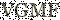# Equation graph plotter - EqPlot for Windows 7 - Eqplot plots 2D graphs of complex equations. - Windows 7 Download# Equation graph plotter - EqPlot 1.3.31

## EqPlot plots 2D graphs from complex equations.

EqPlot plots 2D graphs from complex equations. The application comprises algebraic, trigonometric, hyperbolic and transcendental functions. EqPlot can be used to verify the results of nonlinear regression analysis program. Graphically Review Equations: Up to ten equations could be plotted at the same time, so that intersections and domains could be studied visually.

### Equation graph plotter - EqPlot 1.3.31 full detailsFile Size: 3.37 MB Free To Try \$15.00 2019-02-27 Total: 227 | This Month: 5 Institute of Mathematics and Statistics http://www.math-solutions.orgDownload Equation graph plotter - EqPlot 1.3.31

Save Equation graph plotter - EqPlot to My Stuff

### Equation graph plotter - EqPlot 1.3.31 full description

EqPlot plots 2D graphs from complex equations. The application comprises algebraic, trigonometric, hyperbolic and transcendental functions. EqPlot can be used to verify the results of nonlinear regression analysis program. Graphically Review Equations: EqPlot gives engineers and researchers the power to graphically review equations, by putting a large number of equations at their fingertips. Up to ten equations could be plotted at the same time, so that intersections and domains could be studied visually. Understandable and convenient interface: A flexible work area lets you type in your equations directly. It is as simple as a regular text editor. Annotate, edit and repeat your graphings in the work area. You can also paste your equations into the editor panel. Example of mathematical expression: 5.22 - (2 * x) + square(x) + power(x;3) + power(2.55;4) - logbaseN(4;6.25) Save your work for later use into a text or graphic file. Comprehensive online help is easily accessed within the program. Features: -------- *Scientific graphings *Unlimited expression length *Parenthesis compatible *Scientific notation *More than 35 functions *More than 40 constants *User-friendly error messages *Simple mode (medium size on desktop) *Paste expressions into EqPlot *Comprehensive documentation *All the benefits that Windows bestows, such as multi-tasking and print formatting are available

### Equation graph plotter - EqPlot 1.3.31 Windows 7 release notes

New Release
Higher accuracy and larger extents
[ Equation graph plotter - EqPlot release history ]

### Equation graph plotter - EqPlot 1.3.31 Windows 7 requirements

None### Equation graph plotter - EqPlot for Windows 7 - Copyright information

All Equation graph plotter - EqPlot reviews, submitted ratings and written comments become the sole property of Windows 7 download. You acknowledge that you, not windows7download, are responsible for the contents of your submission. However, windows7download reserves the right to remove or refuse to post any submission for any reason.

Windows 7 Download periodically updates pricing and software information of Equation graph plotter - EqPlot full version from the publisher, but some information may be out-of-date. You should confirm all information.
Using warez version, crack, warez passwords, patches, serial numbers, registration codes, key generator, pirate key, keymaker or keygen for Equation graph plotter - EqPlot license key is illegal and prevent future development of Equation graph plotter - EqPlot. Download links are directly from our mirrors or publisher's website, Equation graph plotter - EqPlot torrent or shared files from free file sharing and free upload services, including Rapidshare, MegaUpload, YouSendIt, SendSpace, DepositFiles, DivShare, HellShare, HotFile, FileServe or MediaFire, are not used.

### Post Equation graph plotter - EqPlot reviewYour Name: Select 1 - Awful 2 - Bad 3 - Usable 4 - Good 5 - Excellent### Windows 7 Equation graph plotter - EqPlot related downloads

... a solution to users who want to create graph paper using a printer. There is a full ...
... a solution to users who want to make graph paper for graphing equations. There are options to ...
The program allows you to solve algebraic equations in the automatic mode. You just enter an ...
This program is for drawing graphs of mathematical functions in a coordinate system. Graphs may be added with different color and line ...
Function Grapher is graph maker to create 2D, 2.5D, 3D and 4D function graphs, animations and table graphs. 2D functions can be ...
My Account
Help
Windows 7 Software Coupons
My Saved Stuff
You have not saved any software.
Click "Save" next to each software.

Would you like to receive announcements of new versions of your software by email or by RSS reader? Register for FREE!